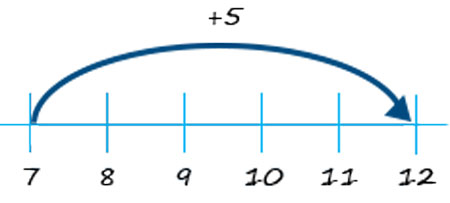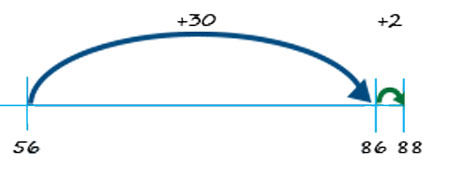# Using a number line for adding on

Take the addition of 7 + 5:

Start at 7, and add on 5 to get 12.Figure 2

Next, the addition of 32 + 56:

Start at 56, add on 30, then 2, and you get 88.Figure 3

2 Using concrete representations to develop students’ understanding

Using a number line for subtraction